# Quantum Phase Transition from Spherical to unstable for

• Slides: 22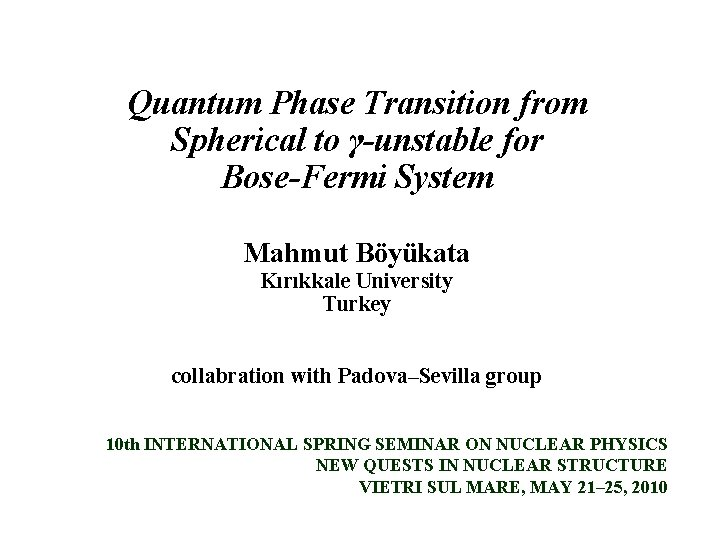Quantum Phase Transition from Spherical to γ-unstable for Bose-Fermi System Mahmut Böyükata Kırıkkale University Turkey collabration with Padova–Sevilla group 10 th INTERNATIONAL SPRING SEMINAR ON NUCLEAR PHYSICS NEW QUESTS IN NUCLEAR STRUCTURE VIETRI SUL MARE, MAY 21– 25, 2010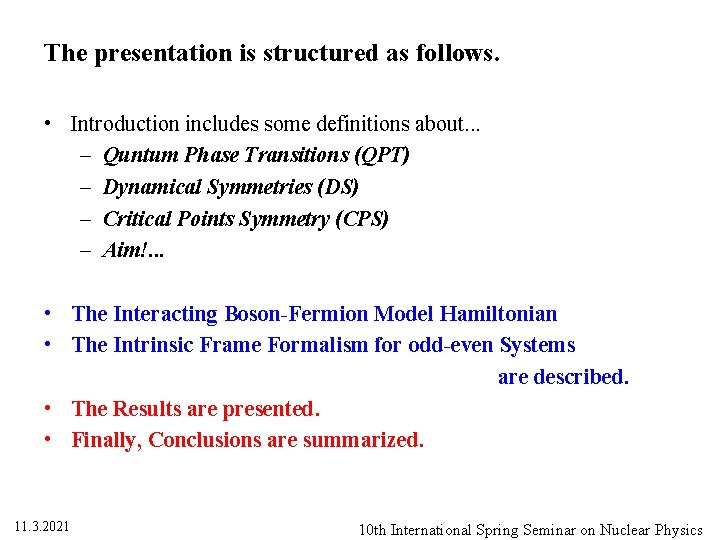The presentation is structured as follows. • Introduction includes some definitions about. . . – Quntum Phase Transitions (QPT) – Dynamical Symmetries (DS) – Critical Points Symmetry (CPS) – Aim!. . . • The Interacting Boson-Fermion Model Hamiltonian • The Intrinsic Frame Formalism for odd-even Systems are described. • The Results are presented. • Finally, Conclusions are summarized. 11. 3. 2021 10 th International Spring Seminar on Nuclear PhysicsQuantum Phase Transitions: • The study of phase transitions has been the subject in many investigations of the nuclear physics. • Phase transitions can be classified into two classes. – First-order phase transition includes two different phases. • Two coexisting minima of the energy surface have the same energy. – The second kind of phase transitions receive the name of continuous (second-order) phase transition. • The phase change occurs very softly in a continuous way from one phase to the other as the control parameters are varied. Figures from Iachello’s Presentation, Istanbul (2010) 11. 3. 2021 10 th International Spring Seminar on Nuclear Physics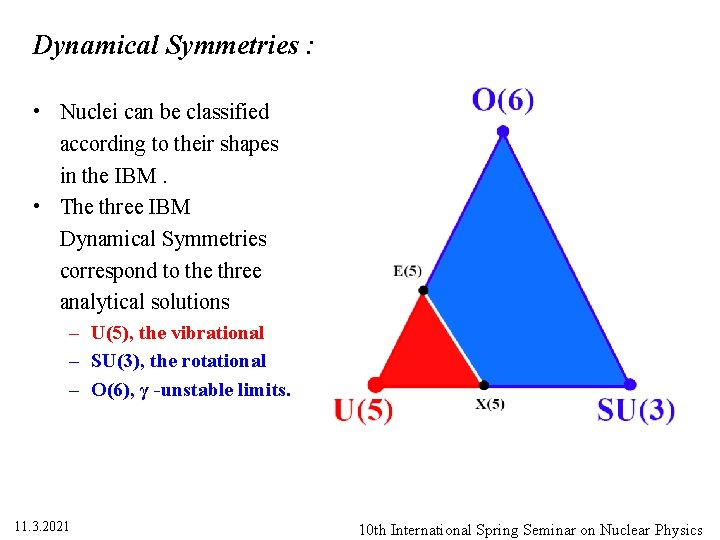Dynamical Symmetries : • Nuclei can be classified according to their shapes in the IBM. • The three IBM Dynamical Symmetries correspond to the three analytical solutions – U(5), the vibrational – SU(3), the rotational – O(6), γ -unstable limits. 11. 3. 2021 10 th International Spring Seminar on Nuclear Physics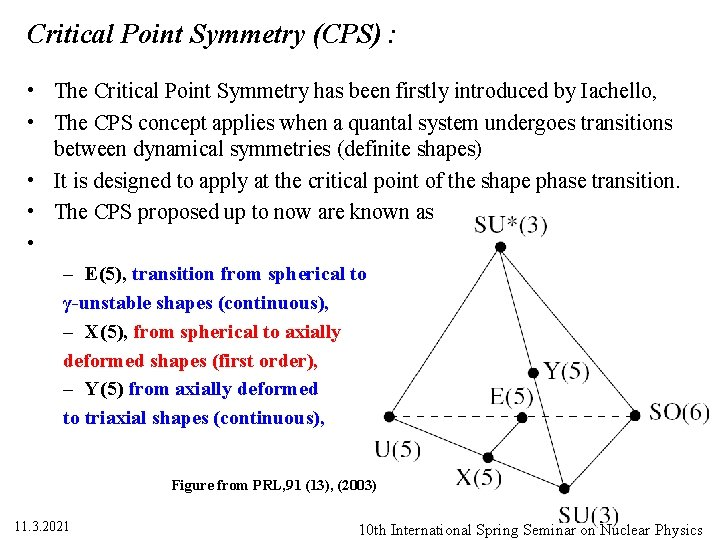Critical Point Symmetry (CPS) : • The Critical Point Symmetry has been firstly introduced by Iachello, • The CPS concept applies when a quantal system undergoes transitions between dynamical symmetries (definite shapes) • It is designed to apply at the critical point of the shape phase transition. • The CPS proposed up to now are known as • – E(5), transition from spherical to γ-unstable shapes (continuous), – X(5), from spherical to axially deformed shapes (first order), – Y(5) from axially deformed to triaxial shapes (continuous), Figure from PRL, 91 (13), (2003) 11. 3. 2021 10 th International Spring Seminar on Nuclear PhysicsDeformed Spherical Oblate c=1 χ = +1, 32 γ-unstable; c=1, χ=0 Spcerical c=0 11. 3. 2021 Prolate c=1 , χ = -1, 32 10 th International Spring Seminar on Nuclear PhysicsPotential Energy Surphase (PES) V(β, γ): • Geometric collective deformations are described by introducing two collective variables, called deformation parameters (β, γ). – The β variable measures the axial deviation from sphericity – The angle variable γ controls the departure from axial deformation. – The β and γ play a similar role to the intrinsic collective shape variables in the Bohr Hamiltonian. 11. 3. 2021 10 th International Spring Seminar on Nuclear Physics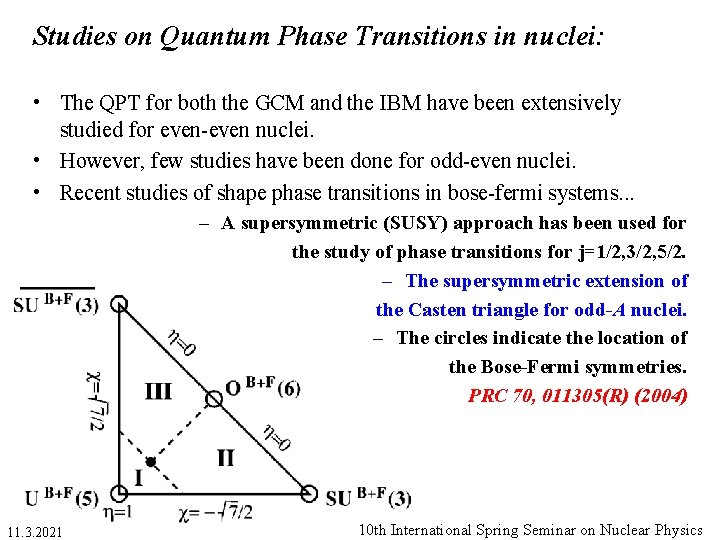Studies on Quantum Phase Transitions in nuclei: • The QPT for both the GCM and the IBM have been extensively studied for even-even nuclei. • However, few studies have been done for odd-even nuclei. • Recent studies of shape phase transitions in bose-fermi systems. . . – A supersymmetric (SUSY) approach has been used for the study of phase transitions for j=1/2, 3/2, 5/2. – The supersymmetric extension of the Casten triangle for odd-A nuclei. – The circles indicate the location of the Bose-Fermi symmetries. PRC 70, 011305(R) (2004) 11. 3. 2021 10 th International Spring Seminar on Nuclear Physics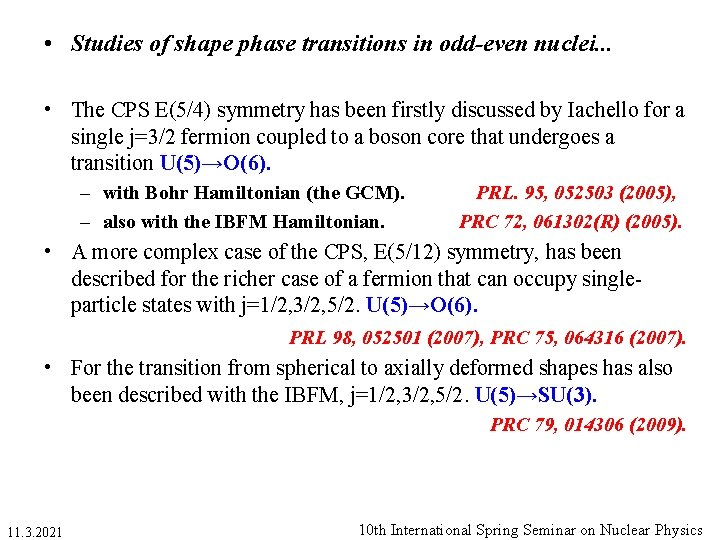• Studies of shape phase transitions in odd-even nuclei. . . • The CPS E(5/4) symmetry has been firstly discussed by Iachello for a single j=3/2 fermion coupled to a boson core that undergoes a transition U(5)→O(6). – with Bohr Hamiltonian (the GCM). – also with the IBFM Hamiltonian. PRL. 95, 052503 (2005), PRC 72, 061302(R) (2005). • A more complex case of the CPS, E(5/12) symmetry, has been described for the richer case of a fermion that can occupy singleparticle states with j=1/2, 3/2, 5/2. U(5)→O(6). PRL 98, 052501 (2007), PRC 75, 064316 (2007). • For the transition from spherical to axially deformed shapes has also been described with the IBFM, j=1/2, 3/2, 5/2. U(5)→SU(3). PRC 79, 014306 (2009). 11. 3. 2021 10 th International Spring Seminar on Nuclear PhysicsIn this study. . . • We focus on the effect of the coupling – a fermion in orbit of definite j – to a boson core that performs the transition U(5)→O(6). • The aim is to understand: – How the coupling of the odd particle modifies the geometry imposed by the core, – How each of the individual coupled states behaves at the transitional region, – How the critical point is affected by the inclusion of the odd particle. • This situation is described with the intrinsic frame formalism for the IBFM. 11. 3. 2021 10 th International Spring Seminar on Nuclear Physics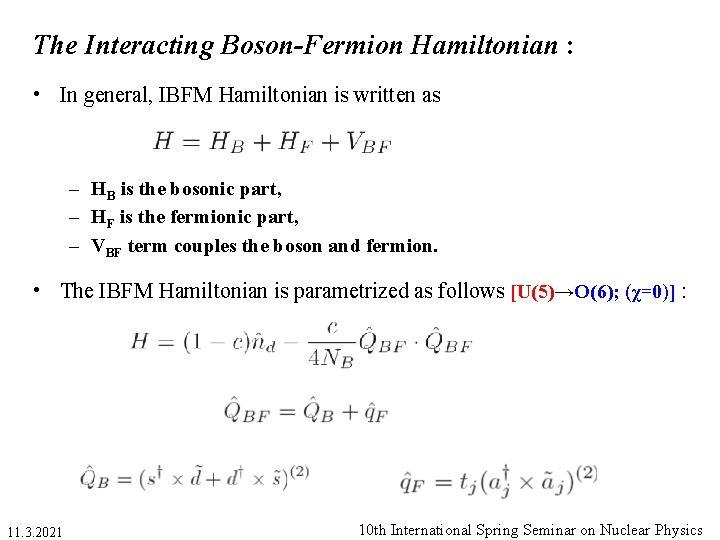The Interacting Boson-Fermion Hamiltonian : • In general, IBFM Hamiltonian is written as – HB is the bosonic part, – HF is the fermionic part, – VBF term couples the boson and fermion. • The IBFM Hamiltonian is parametrized as follows [U(5)→O(6); (χ=0)] : 11. 3. 2021 10 th International Spring Seminar on Nuclear PhysicsThe IBFM Hamiltonian : • The boson Hamiltonian [U(5); c=0 and O(6); c=1, χ=0. ] • By changing c between two limits, a continuous (2 nd-order) transition is observed with the critical point at • The boson-fermion interaction 11. 3. 2021 10 th International Spring Seminar on Nuclear Physics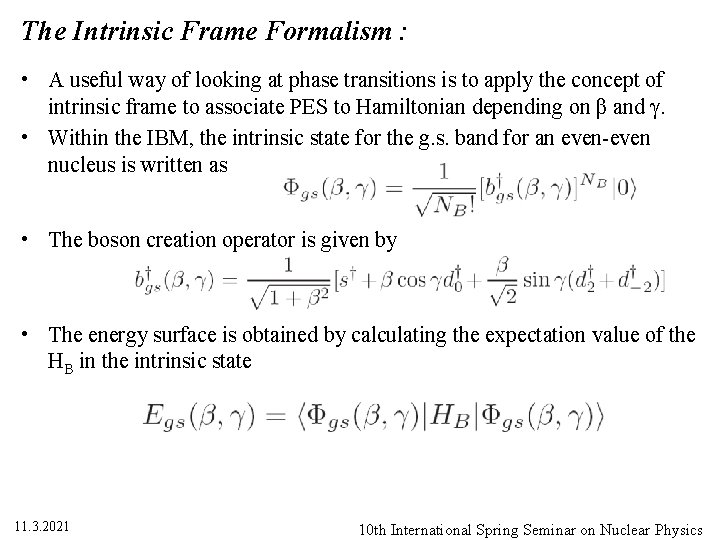The Intrinsic Frame Formalism : • A useful way of looking at phase transitions is to apply the concept of intrinsic frame to associate PES to Hamiltonian depending on β and γ. • Within the IBM, the intrinsic state for the g. s. band for an even-even nucleus is written as • The boson creation operator is given by • The energy surface is obtained by calculating the expectation value of the HB in the intrinsic state 11. 3. 2021 10 th International Spring Seminar on Nuclear PhysicsThe Intrinsic Frame Formalism for odd-even nuclei: • Intrinsic frame states for the mixed boson-fermion system can be constructed by coupling the odd single particle states to the intrinsic states of the boson core. • To obtain them, we first construct the coupled states • Then diagonalize the total boson-fermion Hamiltonian in this basis, giving a set of energy eigenvalues En(β, γ). – Here n is an index to count solutions in the odd-even system. 11. 3. 2021 10 th International Spring Seminar on Nuclear Physics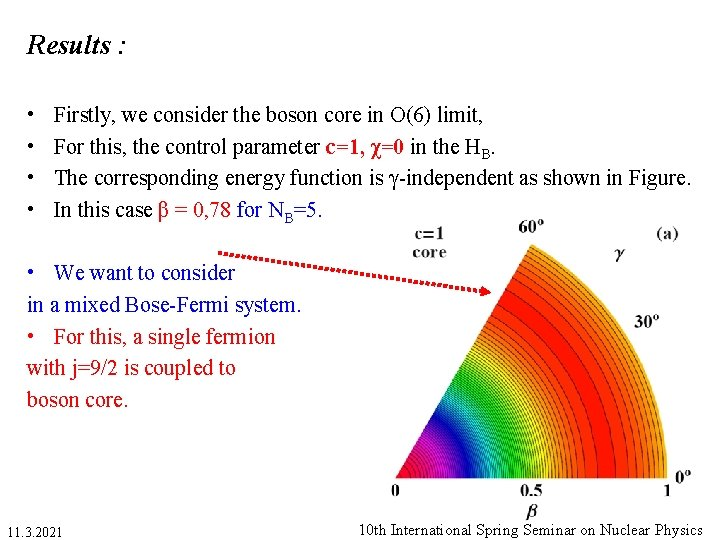Results : • • Firstly, we consider the boson core in O(6) limit, For this, the control parameter c=1, χ=0 in the HB. The corresponding energy function is γ-independent as shown in Figure. In this case β = 0, 78 for NB=5. • We want to consider in a mixed Bose-Fermi system. • For this, a single fermion with j=9/2 is coupled to boson core. 11. 3. 2021 10 th International Spring Seminar on Nuclear Physics• Results : • For j=9/2, the possible magnetic components are K=− 9/2, . . . , +9/2. – There are 10 different states, we restrict to 5 since the symmetry K ↔ -K. • In Fig. (b), the odd-even energy surfaces are plotted as a function of β. • States are labeled by the quantum number K. • The minimum of each surface is marked with a dot. – K=7/2, 9/2 favor oblate shapes – K=1/2, 3/2, 5/2 favor prolate • In Fig. (c) Nilsson-like scheme is obtained when representing the odd-even energy surfaces relative to the energy of the even-even system. 11. 3. 2021 10 th International Spring Seminar on Nuclear Physics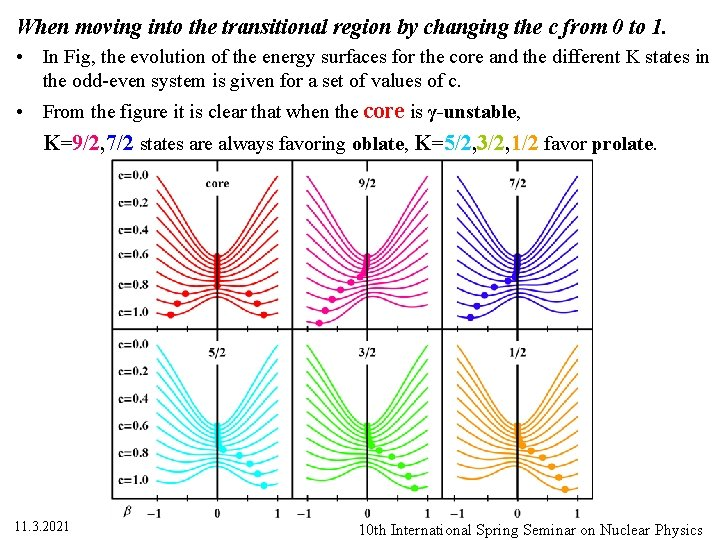When moving into the transitional region by changing the c from 0 to 1. • In Fig, the evolution of the energy surfaces for the core and the different K states in the odd-even system is given for a set of values of c. • From the figure it is clear that when the core is γ-unstable, K=9/2, 7/2 states are always favoring oblate, K=5/2, 3/2, 1/2 favor prolate. 11. 3. 2021 10 th International Spring Seminar on Nuclear Physics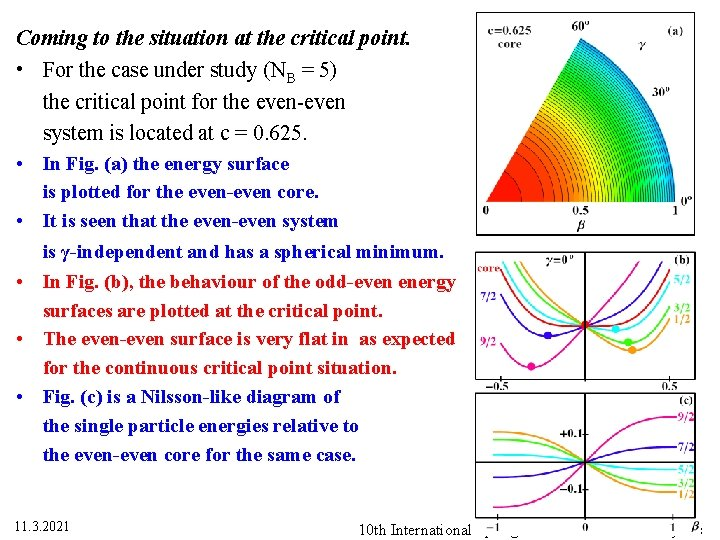Coming to the situation at the critical point. • For the case under study (NB = 5) the critical point for the even-even system is located at c = 0. 625. • In Fig. (a) the energy surface is plotted for the even-even core. • It is seen that the even-even system is γ-independent and has a spherical minimum. • In Fig. (b), the behaviour of the odd-even energy surfaces are plotted at the critical point. • The even-even surface is very flat in as expected for the continuous critical point situation. • Fig. (c) is a Nilsson-like diagram of the single particle energies relative to the even-even core for the same case. 11. 3. 2021 10 th International Spring Seminar on Nuclear PhysicsThe overall results are summarized in Figwhere the minima in for the different odd-even states are plotted versus the control parameter c. • Positive values correspond to prolate deformation, while negative ones mean oblate shapes. • The even-even case is plotted as a reference. • This splits into two lines in the deformed region since for the even-even case two degenerate minima appear. • From the figure one can see that all over the transition the states with K=1/2, 3/2, 5/2 prefer to be prolate while K=7/2, 9/2 are producing oblate shapes. • The upper panel is for NB=5, while the lower one is for NB=15. 11. 3. 2021 10 th International Spring Seminar on Nuclear PhysicsThe overall results are summarized in Figwhere the minima in for the different odd-even states are plotted versus the control parameter c. • In both cases, it is seen that the odd surfaces tend to follow the behaviour of the even-even core. • However, for the smaller NB value, the deviations from the even-even case are larger for all K’s. • As NB grows, the transition of the odd-even system gets closer to the one in the even-even system. • In our case, the γ-instability of the core allows the odd states to drive the entire system towards either a prolate or a oblate shape depending on the value of K. 11. 3. 2021 10 th International Spring Seminar on Nuclear Physics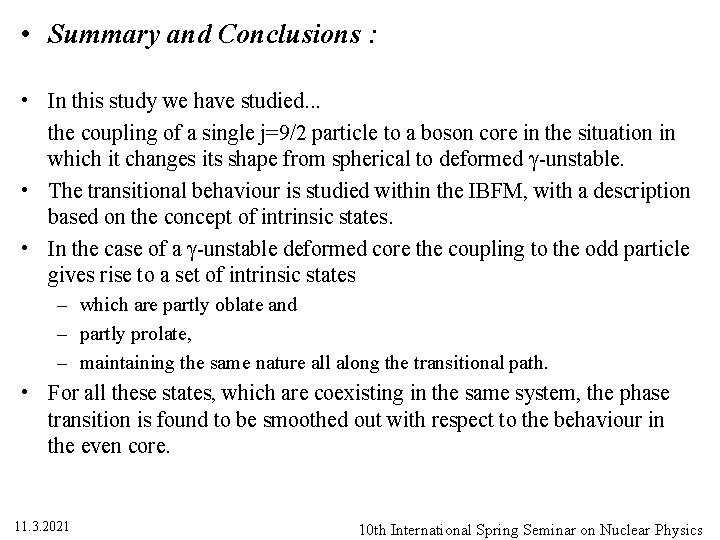• Summary and Conclusions : • In this study we have studied. . . the coupling of a single j=9/2 particle to a boson core in the situation in which it changes its shape from spherical to deformed γ-unstable. • The transitional behaviour is studied within the IBFM, with a description based on the concept of intrinsic states. • In the case of a γ-unstable deformed core the coupling to the odd particle gives rise to a set of intrinsic states – which are partly oblate and – partly prolate, – maintaining the same nature all along the transitional path. • For all these states, which are coexisting in the same system, the phase transition is found to be smoothed out with respect to the behaviour in the even core. 11. 3. 2021 10 th International Spring Seminar on Nuclear PhysicsCOLLABORATORS C. E. Alonso, J. M. Arias L. Fortunato A. Vitturi Sevilla University, Spain ECT*, Trento, Italy Padova University, Italy WE ACKNOWLEDGMENTS F. Iachello A. Leviatan D. Petrellis for many useful discussions on this study.